# 6 th Grade Solving Equations with Multiplication Division

• Slides: 33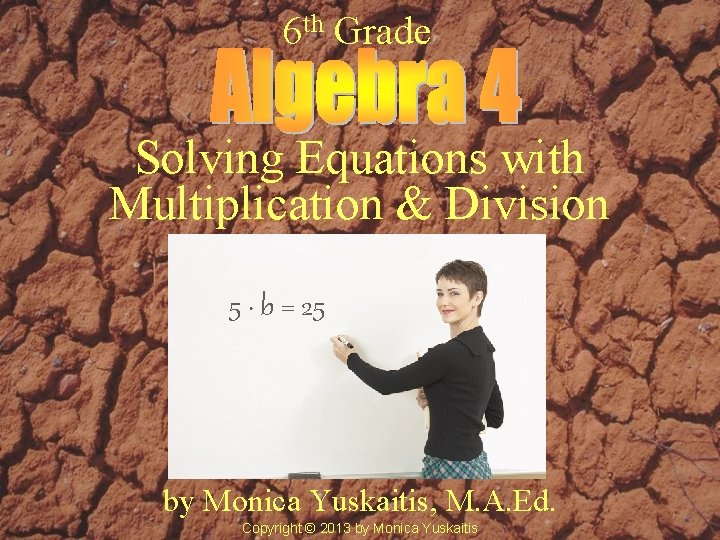6 th Grade Solving Equations with Multiplication & Division 5 · b = 25 by Monica Yuskaitis, M. A. Ed. Copyright © 2013 by Monica Yuskaitis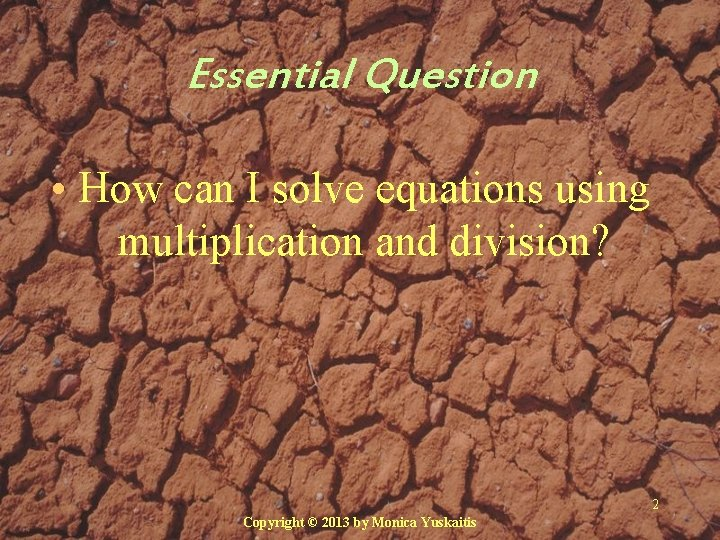Essential Question • How can I solve equations using multiplication and division? 2 Copyright © 2013 by Monica YuskaitisVocabulary 3Vocabulary • product – the answer to a multiplication problem. • factor – a number that is multiplied in a multiplication problem. 2 x 6 = 12 factor product Copyright © 2013 by Monica YuskaitisVocabulary • divisor – a number that divides into another. • dividend – a number that the divisor is divided into. • quotient – the answer to a division problem. 3 quotient divisor 2 ) 6 dividend Copyright © 2013 by Monica Yuskaitis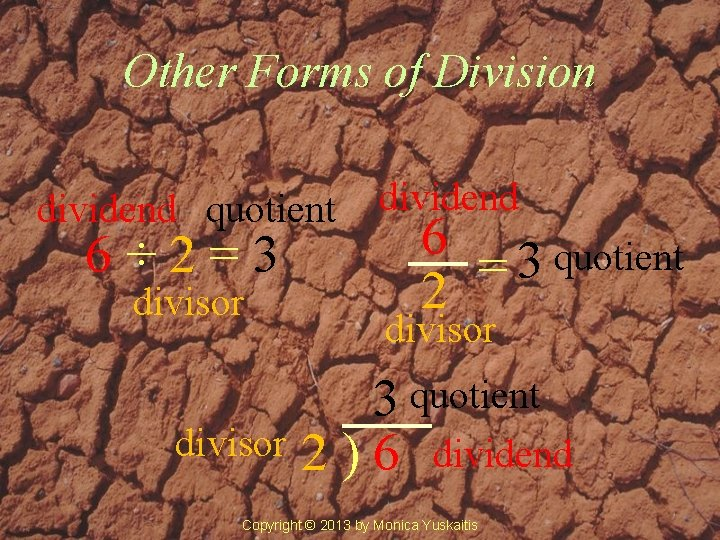Other Forms of Division dividend quotient 6÷ 2=3 divisor dividend 6 3 quotient = 2 divisor 3 quotient divisor 2 ) 6 dividend Copyright © 2013 by Monica YuskaitisVocabulary • Equation – A mathematical sentence stating that 2 expressions are equal. • 8 x 3 = 24 • 30 ÷ 3 = 10 Copyright © 2013 by Monica YuskaitisVocabulary • Equation – A mathematical sentence with an equals sign. • 16 ÷ 2 = 8 • 56 = 7 x 8 Copyright © 2013 by Monica Yuskaitis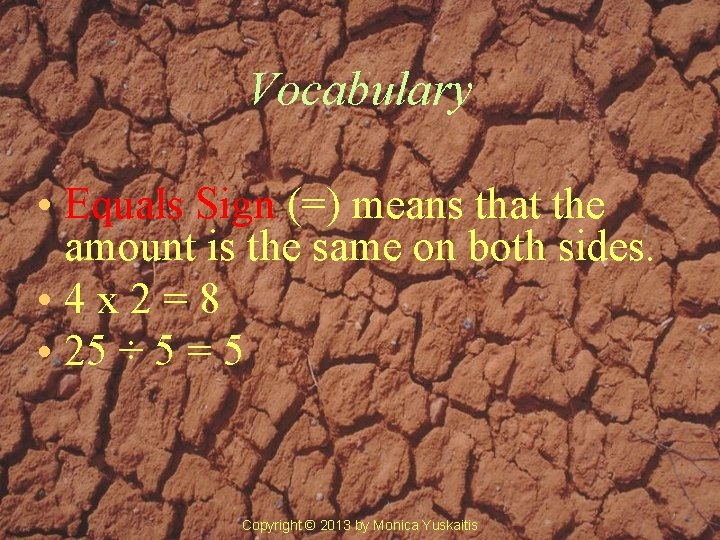Vocabulary • Equals Sign (=) means that the amount is the same on both sides. • 4 x 2=8 • 25 ÷ 5 = 5 Copyright © 2013 by Monica Yuskaitis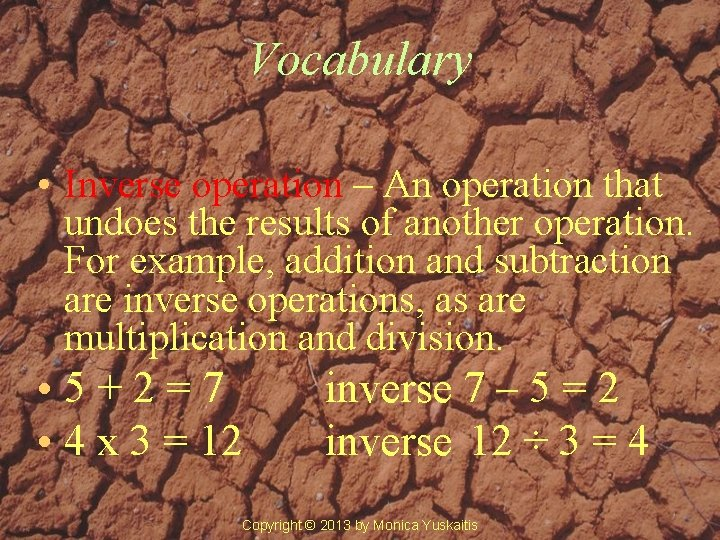Vocabulary • Inverse operation – An operation that undoes the results of another operation. For example, addition and subtraction are inverse operations, as are multiplication and division. • 5+2=7 • 4 x 3 = 12 inverse 7 – 5 = 2 inverse 12 ÷ 3 = 4 Copyright © 2013 by Monica Yuskaitis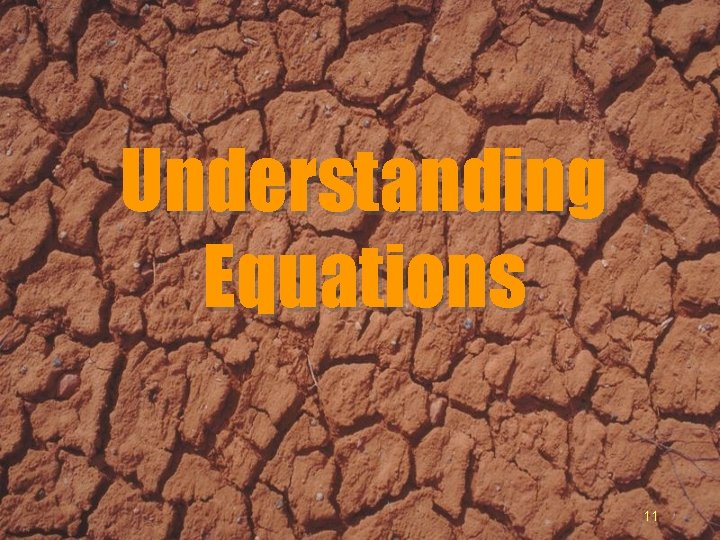Understanding Equations 11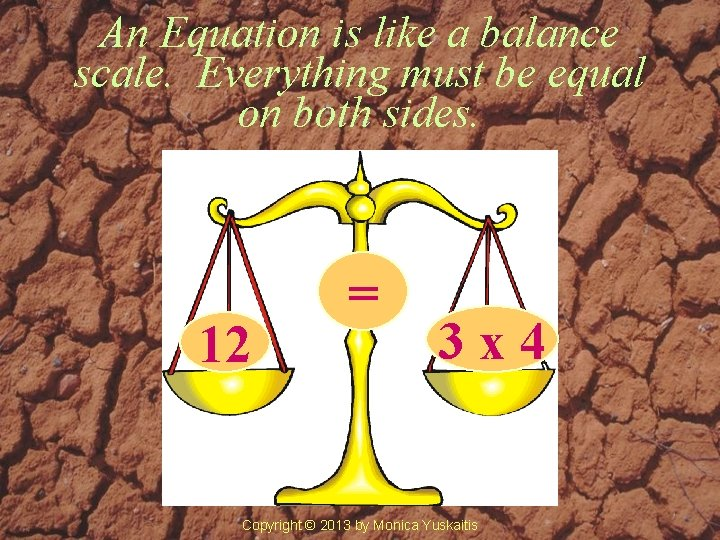An Equation is like a balance scale. Everything must be equal on both sides. 12 = 3 x 4 Copyright © 2013 by Monica Yuskaitis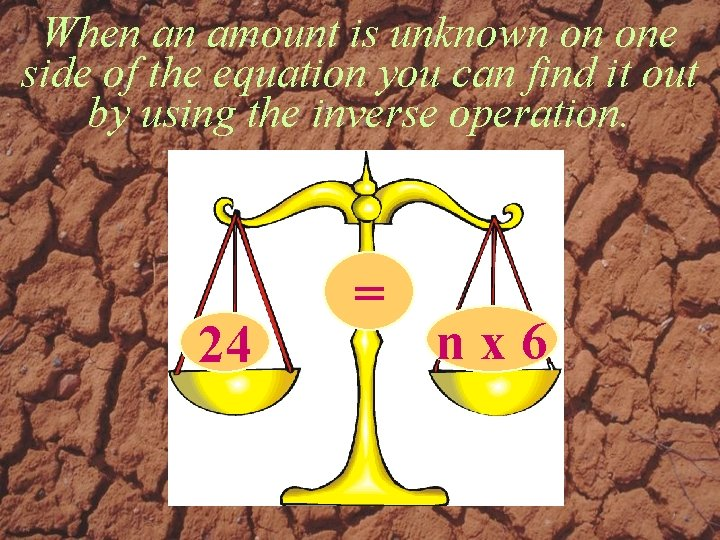When an amount is unknown on one side of the equation you can find it out by using the inverse operation. 24 = nx 6The inverse operation of multiplication is division. Divide both sides by 6 to find n. 24 6 = nx 6 6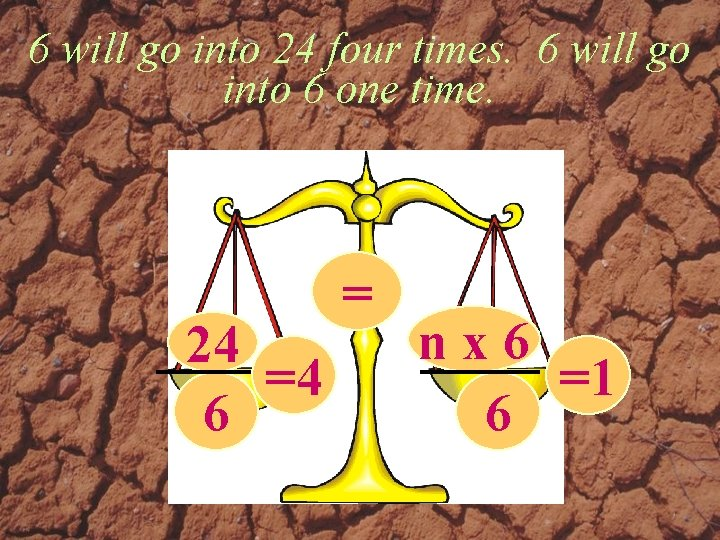6 will go into 24 four times. 6 will go into 6 one time. 24 =4 6 = nx 6 =1 61 times n equals n 4 = nx 1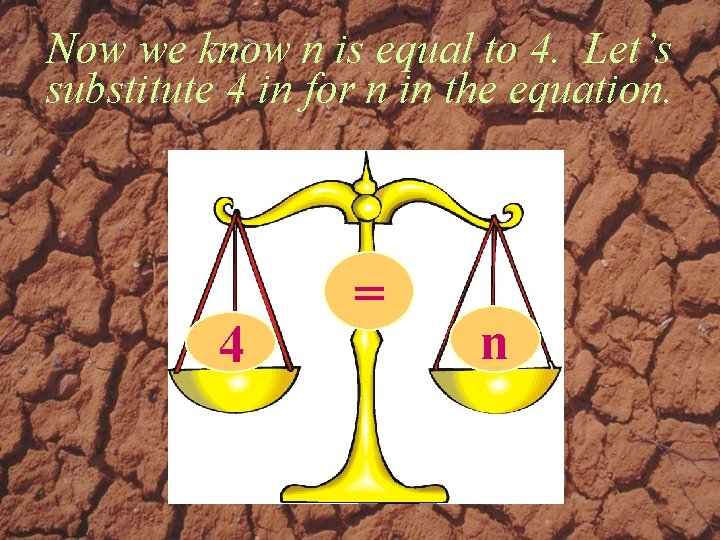Now we know n is equal to 4. Let’s substitute 4 in for n in the equation. 4 = n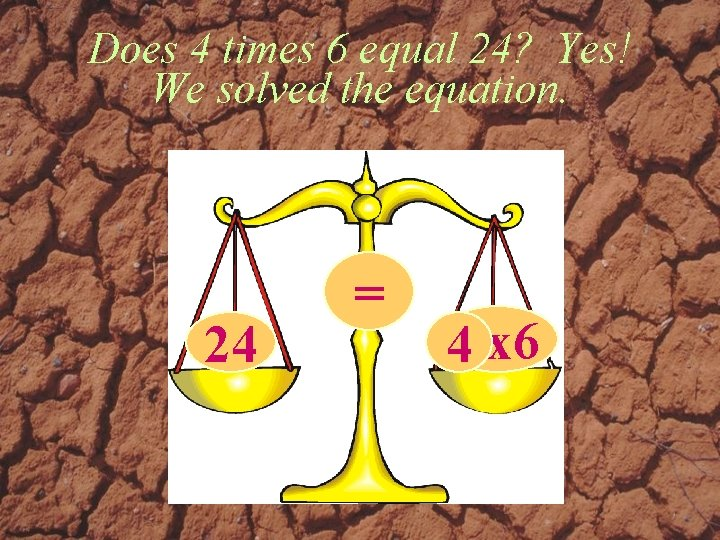Does 4 times 6 equal 24? Yes! We solved the equation. 24 = 4 nx 6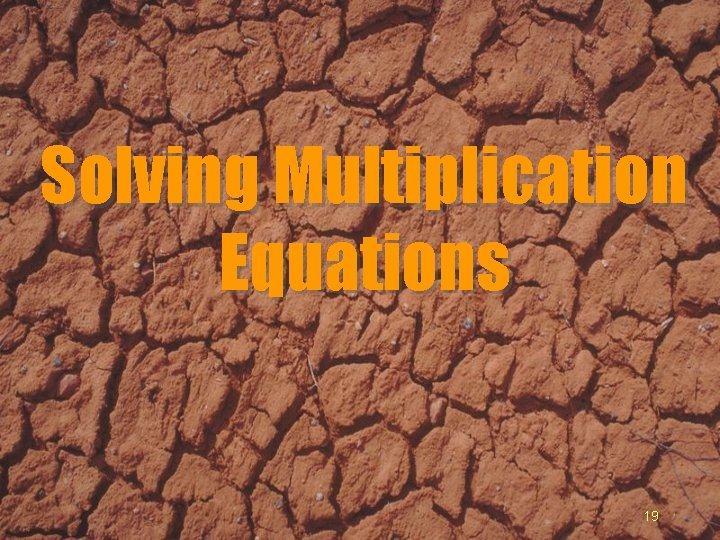Solving Multiplication Equations 19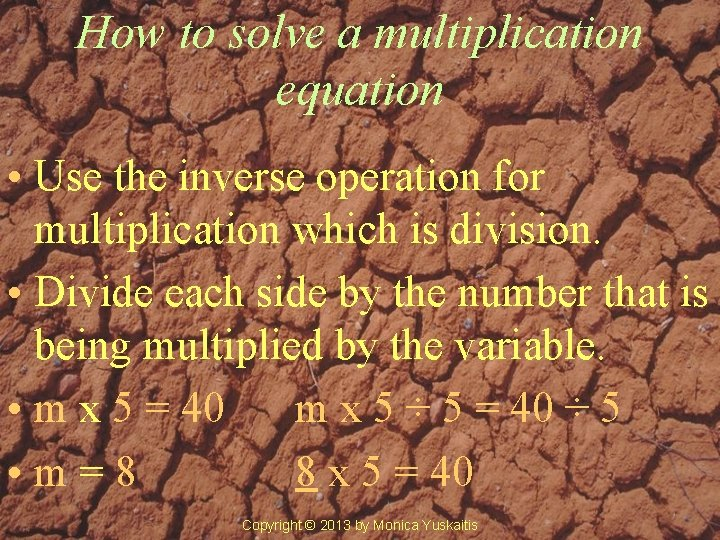How to solve a multiplication equation • Use the inverse operation for multiplication which is division. • Divide each side by the number that is being multiplied by the variable. • m x 5 = 40 m x 5 ÷ 5 = 40 ÷ 5 • m=8 8 x 5 = 40 Copyright © 2013 by Monica YuskaitisNow it’s your turn to solve the problems. Copyright © 2013 by Monica Yuskaitis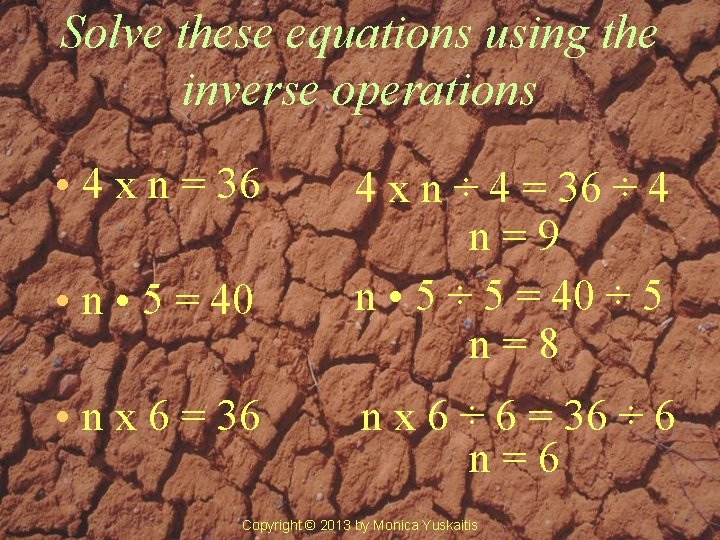Solve these equations using the inverse operations • 4 x n = 36 • n • 5 = 40 • n x 6 = 36 4 x n ÷ 4 = 36 ÷ 4 n=9 n • 5 ÷ 5 = 40 ÷ 5 n=8 n x 6 ÷ 6 = 36 ÷ 6 n=6 Copyright © 2013 by Monica Yuskaitis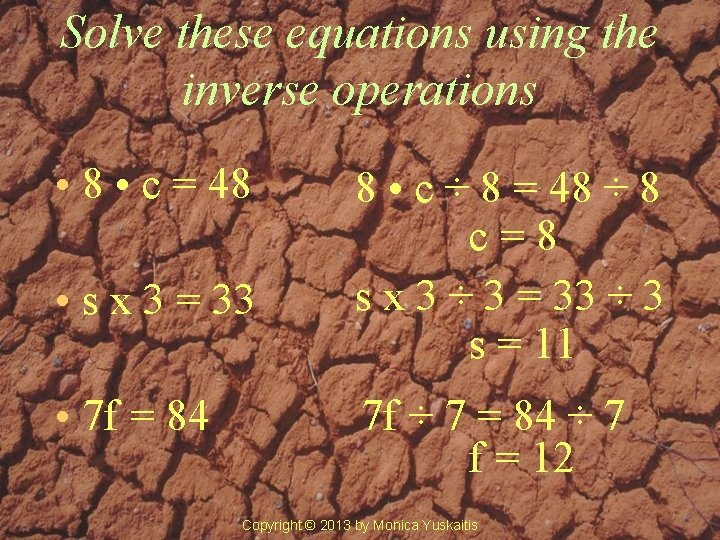Solve these equations using the inverse operations • 8 • c = 48 • s x 3 = 33 • 7 f = 84 8 • c ÷ 8 = 48 ÷ 8 c=8 s x 3 ÷ 3 = 33 ÷ 3 s = 11 7 f ÷ 7 = 84 ÷ 7 f = 12 Copyright © 2013 by Monica YuskaitisSolving Division Equations 24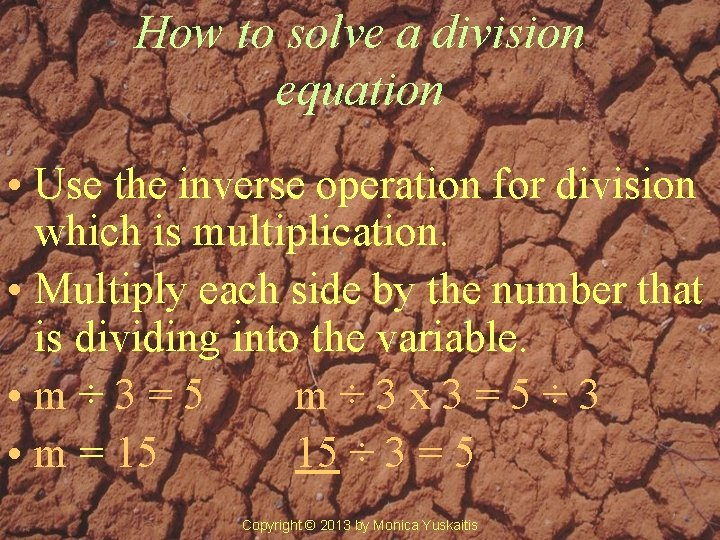How to solve a division equation • Use the inverse operation for division which is multiplication. • Multiply each side by the number that is dividing into the variable. • m÷ 3=5 m÷ 3 x 3=5÷ 3 • m = 15 15 ÷ 3 = 5 Copyright © 2013 by Monica Yuskaitis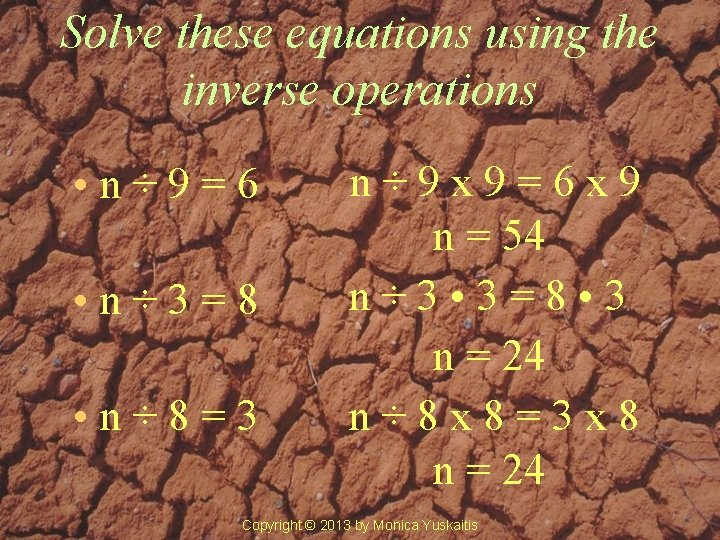Solve these equations using the inverse operations • n÷ 9=6 • n÷ 3=8 • n÷ 8=3 n÷ 9 x 9=6 x 9 n = 54 n÷ 3 • 3=8 • 3 n = 24 n÷ 8 x 8=3 x 8 n = 24 Copyright © 2013 by Monica YuskaitisSolve these equations using the inverse operations • d÷ 5=5 • r÷ 6=7 • y÷ 7=4 d÷ 5 x 5=5 x 5 d = 25 r÷ 6 x 6=7 x 6 r = 42 y÷ 7 • 7=4 • 7 y = 28 Copyright © 2013 by Monica Yuskaitis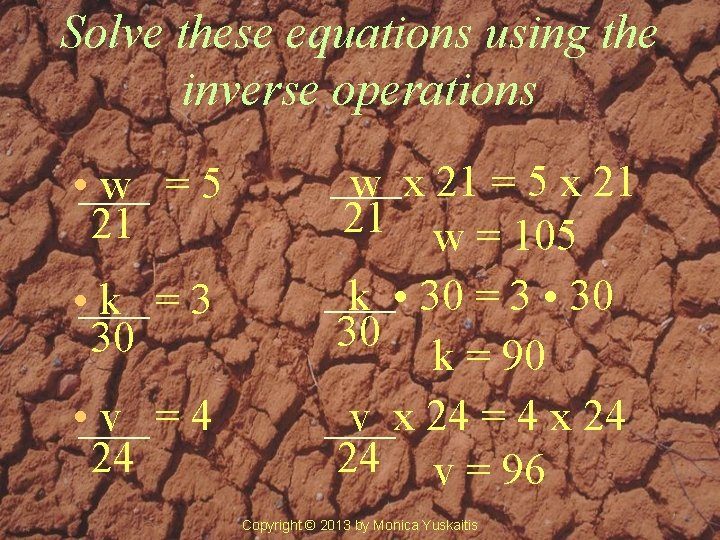Solve these equations using the inverse operations • w =5 21 • k =3 30 • v =4 24 w x 21 = 5 x 21 21 w = 105 k • 30 = 3 • 30 30 k = 90 v x 24 = 4 x 24 24 v = 96 Copyright © 2013 by Monica YuskaitisProblem Solving 29The Science Museum FieldtripProblem Solving Steps What information do you need? What is the problem asking you to find out? Is the answer going to be larger or smaller? What strategies will you use? What operations will you use? How many steps are there and in what order must you do them? Analysis Representation How will you solve the problem? Did you check your answer? Does your answer make sense? ResolutionThe sixth grades went to the Academy of Science Museum in San Francisco. There were 3 buses and each bus held 42 passengers. How many went on the field trip? n 3· 3 = 42 · 3 n = 126This is for you for working so hard! Copyright © 2013 by Monica Yuskaitis